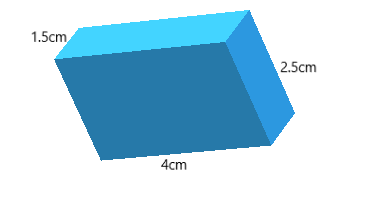QUESTION

# A match box measures $4cm \times 2.5cm \times 1.5cm$. What will be the volume of a packet that contains 12 match boxes?

Hint: Here, we need to find the volume of 1 match box, we will use the volume of box formula i.e. $V = l \times b \times h$ and then we need to calculate the volume of the packet that contains 12 match boxes.

Generally any box measure will be of $l,b,h$
Given that a match box measures $4cm \times 2.5cm \times 1.5cm$So here $l = 4cm,b = 2.5cm,h = 1.5cm$
We know that volume of box $(v) = l \times b \times h$
$\therefore$ Volume of a match box= $4cm \times 2.5cm \times 1.5cm = 15c{m^3}$
We know that volume of $1$ match box =$15c{m^3}$
Then volume of $12$ matchboxes=$12 \times 15$
$\therefore$ Volume of $12$ match boxes = $180c{m^3}$
Note: Units are mandatory. Here the units for the volume of the box is $c{m^3}$. Make sure that all the measures are of the same unit, if not convert all the measures into the same unit by using basic metric conversions.